# RRB Clerk Prelims Quantitative Aptitude Questions 2021 (Day-10)

Dear Aspirants, Our IBPS Guide team is providing new series of Quantitative Aptitude Questions for RRB Clerk Prelims 2021 so the aspirants can practice it on a daily basis. These questions are framed by our skilled experts after understanding your needs thoroughly. Aspirants can practice these new series questions daily to familiarize with the exact exam pattern and make your preparation effective.

Start Quiz

Profit and loss

1) Mr. Sharma purchased a smart television for Rs 50000. Its price was marked up by 20%. He sold the television at a discount of 10% on the marked price. What is the profit percentage on the cost price?

A.3%

B.7%

C.5%

D.8%

E.None of these

Time and work

2) A, B and C together can complete the work in 16 days. If A, B and C started the work together, after 4 days A is left the work and then C and B together can complete the remaining work in 24 days. In how many days A alone complete the work?

A.32 days

B.24 days

C.30 days

D.27 days

E.36 days

Partnership

3) A and B started a business with an investment of Rs 1400 and Rs 2700 respectively. After 6 months, C joined with Rs 2400. If the difference between A’s share and C’s share in the annual profit was Rs 400, what was the annual profit?

A.15000

B.10600

C.10300

D.10100

E.None of these

Boat and stream

4) A motorboat goes 12 km upstream in 48 minutes. If the speed of the stream is 1/6 of the speed of the boat in still water, then how much distance (in km) can the motorboat travel downstream in 40 minutes?

A.17 km

B.14 km

C.19 km

D.31 km

E.None of these

Mensuration

5) The difference between the area of a rectangle and a square is 70 cm2. If the rectangle’s breadth and length 20% less and 25% more than the side of the square respectively, then find the area of rectangle

A.216

B.36

C.256

D.Can’t be determined

E.None of these

Simplification

Directions (06-10): What will come in place of question mark in the following questions?

6)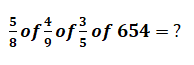A.111

B.113

C.99

D.121

E.109

7)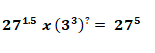A.3.5

B.1.3

C.2.5

D.1.5

E.3.3

8) 28.06 x 25 – ? = 260

A.343.6

B.256.8

C.216.4

D.441.5

E.512.5

9) 56% of 590+ ?=605

A.274.6

B.280.5

C.286.5

D.296.4

E.275.4

10)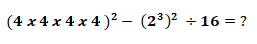A.65536

B.62485

C.54785

D.65532

E.65526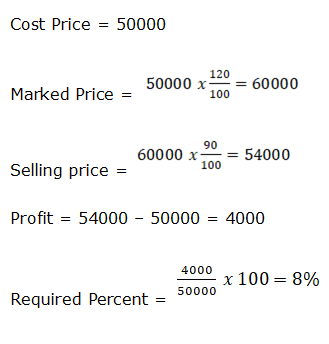A, B and C together can complete the work in 4 days = 4/16 = ¼

Remaining work = 1 – ¼ = ¾

C and B together can complete the whole work = 4/3 * 24 = 32 days

A alone complete the work = 1/16 – 1/32 = 1/32

Ratio of profit of A:B:C

1400 x 12: 2700 x 12: 2400 x 6

14 :27: 12

Now, let the profit of A, B and C be 14X, 27X and 12X respectively.

Then, 14X – 12X = 400

X = 200

Total profit = 14X + 27X + 12X = 53X

= 53 x 200 = 10600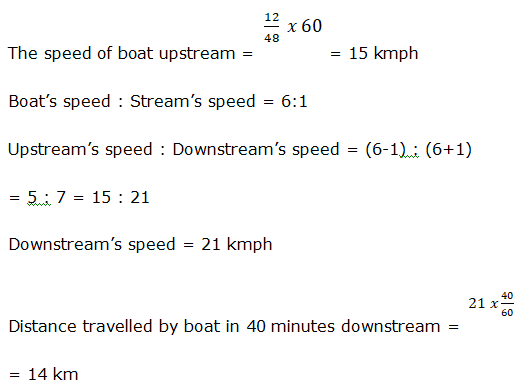Side of the square be a cm.

Breadth of the rectangle = 0.8a

Length of the rectangle = 1.25a

Now,

1.25a x 0.8a – a2= 70

a2 – a= 70

Here we can’t find the side of square.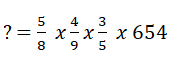? = 109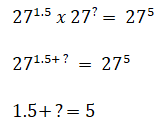? = 5 -1.5

? = 3.5

? = 28.06 x 25 – 260

? = 701.5 – 260

? = 441.5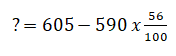? = 605 – 330.4

? = 274.6